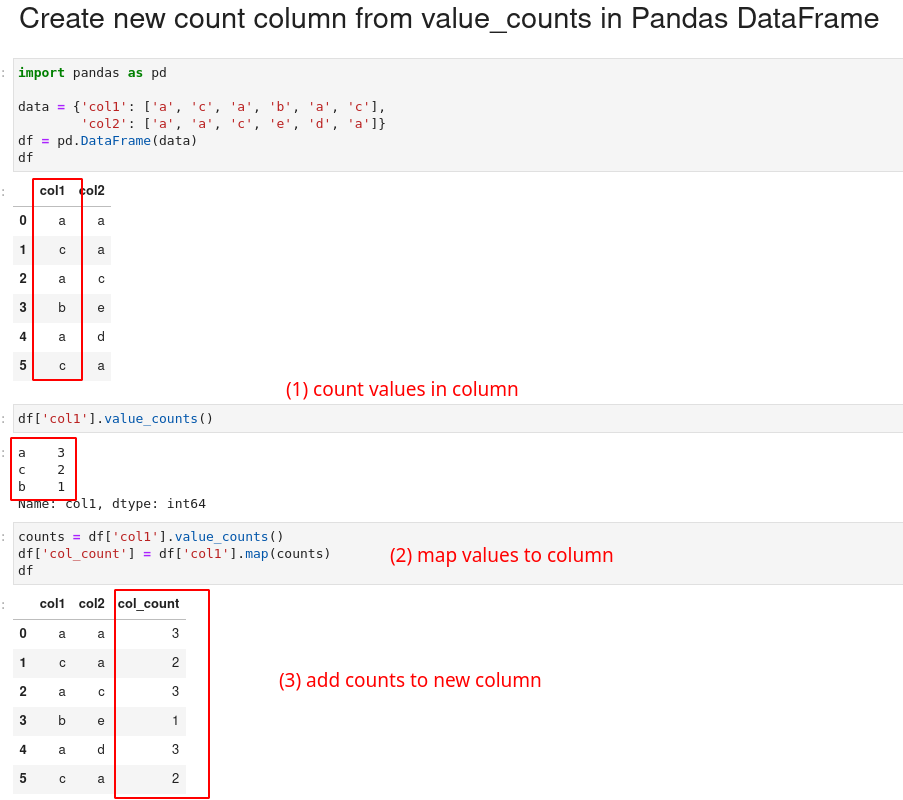In this short guide, I'll show you how to create a new count column based on `value_counts` from another column in Pandas DataFrame.

There are multiple ways to count values and add them as new column:

(1) value_counts and map

``````counts = df['col1'].value_counts()
df['col_count'] = df['col1'].map(counts)
``````

(2) group by and transform

``````df['col_count'] = df.groupby(['col1'])['col1'].transform('count')
``````

You can also read the tricky related topic: How to Group by multiple columns, count and map in Pandas .

• How do I count values in a new column in pandas?
• How do I create a new column based on another column value in pandas?
• How do I count values in one column based on another column?

Let's discuss the advantages and disadvantages of both of them in a few examples.## Setup

Let's create a sample DataFrame to count values in it's columns:

``````import pandas as pd

data = {'col1': ['a', 'c', 'a', 'b', 'a', 'c'],
'col2': ['x', 'y', 'z', 'x', 'x', 'y']}
df = pd.DataFrame(data)
``````

DataFrame looks like:

col1 col2
0 a a
1 c a
2 a c
3 b e
4 a d
5 c b

## value_counts and map to column

I prefer to use value_counts and then map the counts to a given column. Finally we assign the values to new column:

``````counts = df['col1'].value_counts()
df['col_count'] = df['col1'].map(counts)
``````

we can write the same in a single line:

``````df['col_count'] = df['col1'].map(df['col1'].value_counts())
``````

result:

col1 col2 col_count
0 a a 3
1 c a 2
2 a c 3
3 b e 1
4 a d 3
5 c b 2

The advantage of this way is that we can map to different column and it's easier to read.

How does it work?

• the method `value_counts` calculates the count of unique values in the column `col1`.
• next `map` function maps the values in `col1` to the corresponding count in the resulting Series.
• the result is then assigned to a new column `col_count` in the DataFrame.

## Count and map to another column

We can count values in column `col1` but map the values to column `col2`.

``````counts = df['col1'].value_counts()
df['col_count'] = df['col2'].map(counts)
``````

This time count is mapped to `col2` but the count is based on `col1`. This is very useful when we work with child-parent relationship:

col1 col2 col_count
0 a a 3.0
1 c a 3.0
2 a c 2.0
3 b e NaN
4 a d NaN
5 c b 1.0

## group by and transform

In this section we will discuss how to add a counter per group in Pandas. We can group by one column, count and then transform the results to new column:

``````df['col_count'] = df.groupby(['col1'])['col1'].transform('count')
``````

We get the same result as before:

col1 col2 col_count
0 a a 3
1 c a 2
2 a c 3
3 b e 1
4 a d 3
5 c b 2

## Performance comparison

There's no difference in mid size DataFrames for both approaches:

• `df.groupby(['col1'])['col1'].transform('count')`
• 1.12 ms ± 15.2 µs per loop (mean ± std. dev. of 7 runs, 1000 loops each)
• `df['col1'].value_counts();df['col_count'] = df['col1'].map(counts)`
• 1.15 ms ± 35.6 µs per loop (mean ± std. dev. of 7 runs, 1000 loops each)

Tests were done with 6000 rows.

## Conclusion

In this article, we saw how to use Pandas `groupby` and `value_counts` to add a new count column in DataFrame.

We also discussed how to count one column and map counts on another.